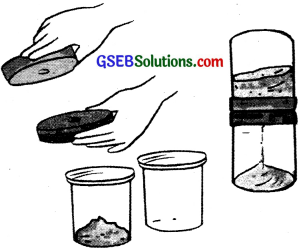# GSEB Solutions Class 7 Science Chapter 13 Motion and Time

Gujarat Board GSEB Textbook Solutions Class 7 Science Chapter 13 Motion and Time Textbook Questions and Answers, Notes Pdf.

## Gujarat Board Textbook Solutions Class 7 Science Chapter 13 Motion and Time

### Gujarat Board Class 7 Science Motion and Time Textbook Questions and Answers

Question 1.
Classify the following as motion along a straight line, circular or oscillatory motion:

1. The motion of your hands while running.
2. The motion of a horse pulling a cart on a straight road.
3. The motion of a child in a merry-go-round,
4. The motion of a child on a see-saw.
5. The motion of the hammer of an electric bell.
6. The motion of a train on a straight bridge.

1. Oscillatory motion
2. Linear motion
3.  Circular motion
4. Oscillatory motion
5. Oscillatory motion
6. Linear motionQuestion 2.
Which of the following are not correct?
(i) The basic unit of time is second.
(ii) Every object moves at a constant speed.
(iii) Distances between two cities are measured in kilometers.
(iv) The time period of a given pendulum is not constant.
(v) The speed of a train is expressed in m/h.
(ii), (ii), (v)

Question 3.
A simple pendulum takes 32 s to complete 20 oscillations, what is the time period of the pendulum?
Time taken to complete 20 oscillations = 32 s
Time taken to complete 1 oscillation = $$\frac {32}{20}$$ s = 1.6 s
The time period of a pendulum is the time taken by it to complete 1 oscillation. The time period of the pendulum is 1.6 seconds.

Question 4.
The distance between the two stations is 240 km. A train takes 4 hours to cover this distance. Calculate the speed of the train.
Distance = 240 km
Time is taken = 4 hours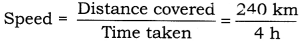= 60 km/h
Speed of train = 60 km/hQuestion 5.
The odometer of a car reads 57321.0 km when the clock shows the time 08:30 AM. What is the distance moved by car, if at 08:50 AM, the odometer reading has changed to 57336.0 km? Calculate the speed of the car in km/ min during this time. Express the speed in km/h also.
Distance = 57336.0 km – 57321.0 km = 15 km
Speed in km/min
$$\frac {15 km}{20 km}$$ = $$\frac {3}{4}$$ km/min
Speed in km/hr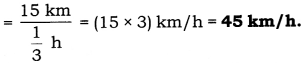Question 6.
Salma takes 15 minutes from her house to reach her school on a bicycle. If the bicycle has a speed of 2 m/min, calculate the distance between her house and the school.
Time is taken =15 min
Speed = 2 m/min
Distance = speed x time = 2 x 15 = 30m
The distance between Salma’s school and her house is 30 m.Question 7.
Show the shape of the distance-time graph for the motion in the following cases:
(i) A car moving at a constant speed.
(ii) A car parked on a side road.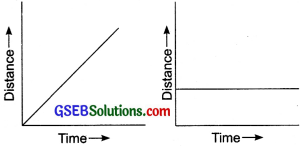Question 8.
Which of the following relations is correct?
(i) Speed = Distance * Time
(ii) Speed = Distance/ Time
(iii) Speed = Time / Distance
(iv) Speed = 1 / Distance x Time
Speed = Distance/Time is correct.

Question 9.
The basic unit of speed is:
(i) km/min
(ii) m/min
(iii) km/h
(iv) m/s
(iv) m/sQuestion 10.
A car moves with a speed of 40 km/h for 15 minutes and then with a speed of 60 km/h for the next 15 minutes. The total distance covered by the car is:
(i) 100 km
(ii) 25 km
(iii) 15 km
(iv) 10 km
(ii) 25 km

Question 11.
Suppose the two photographs, shown in fig. 13.1 and fig. 13.2 of NCERT had been taken at an interval of 10 seconds. If a distance of 100 meters is shown by 1 cm in these photographs, calculate the speed of the fastest car.
The green car is the fastest and it travels 350 m in 10s. So the speed of the car is 135 m/s.

Question 12.
This figure shows the distance-time graph for the motion of two vehicles A and B. Which one of them is moving faster?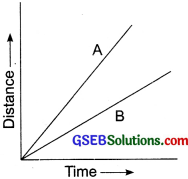‘A’ is moving faster.

Question 13.
Which of the following distance-time graphs shows a truck moving with a speed which is not constant?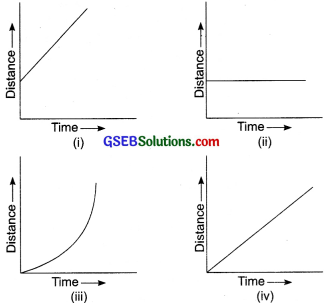Graph (iii)Extended Learning Activities And Projects

Question 1.
You can make your own sundial and use it to mark the time of the day at your place. First of all, find the latitude of your city with the help of an atlas. Cut out a triangular piece of cardboard such that it’s one angle is equal to the latitude of your place and the angle opposite to it is a right angle. Fix this piece, called a gnomon, ‘ vertically along a diameter of a circular board as shown in Fig. One way to face the gnomon could be to make a groove along a diameter on the circular board.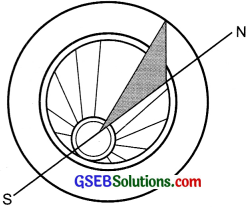Next, select an open space that receives sunlight for most of the day. Mark a line on the ground along the North-South direction. Place the sundial in the sun as shown in Fig. 13.12. Mark the position of the tip of the shadow of the gnomon on the circular board as early in the day as possible, say 8:00 AM. Mark the position of the tip of the shadow every hour throughout the day.

Draw lines to connect each point marked by you with the center of the base of the gnomon as shown in Fig. 13.12. Extend the lines on the circular board up to its periphery. You can use this sundial to read the time of the day at your place. Remember that the gnomon should always be placed in the North-South direction as shown in Fig. 13.12.
The students prepare gnomon and find out the time at any place with the help of gnomon themselves or with the help of their friends.Question 2.
Collect information about time¬measuring devices that were used in ancient times in different parts of the world. Prepare a brief write up on each one of them. The write up may include the name of the device, the place of its origin, the period when it was used, the unit in which the time was measured by it, and a drawing or a photograph of the device, if available.
The time measuring devices used in ancient days are:
1. Sundial: It is situated at Jantar Mantar in Delhi.
2. Sand clock.
3. Water clock.
Students collect various information from various sources with the help of teachers, parents, and newspapers themselves.

Question 3.
Make a model of a sand clock which can measure a time interval of 2 minutes